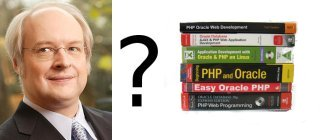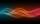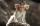Ladislav was reward and went to conference in Amsterdam. Conferense fee was € 3484.

Calculate how many books Ladislav could buy at the price of 48 and 52 euros if he don't want fill whole home library and he can buy only 70 books?

Correct result:

books by € 48:  39
books by € 52:  31

#### Solution:

x+y=70
48•x+52•y=3484

x+y = 70
48x+52y = 3484

x = 39
y = 31

Calculated by our linear equations calculator.Our examples were largely sent or created by pupils and students themselves. Therefore, we would be pleased if you could send us any errors you found, spelling mistakes, or rephasing the example. Thank you!

Please write to us with your comment on the math problem or ask something. Thank you for helping each other - students, teachers, parents, and problem authors.Tips to related online calculators
Do you have a linear equation or system of equations and looking for its solution? Or do you have quadratic equation?

## Next similar math problems:

• MistakeNicol mistake when calculate in school. Instead of add number 20 subtract it. What is the difference between the result and the right result?
• Job applicantsJob applicants: three-fourths of applicants had experience for a position. The number that did not have prior experience was 36. How many people applied for the job?
• Positive integersSeveral positive integers are written on the paper. Michaella only remembered that each number was half the sum of all the other numbers. How many numbers could be written on paper?
• SimplifySimplify expression - which expression is equivalent to: 3(m + 2) − 4(2m − 9)
• IntegerFind the integer whose distance on the numerical axis from number 1 is two times smaller as the distance from number 6.
• EquatiomSolve equation with negatives: X/(-5) + 2 = -9
• Candy and boxesWe have some number of candy and empty boxes. When we put candies in boxes of ten, there will be 2 candies and 8 empty boxes left, when of eight, there will be 6 candies and 3 boxes left. How many candy and empty boxes left when we put candies in boxes of
• Mysterious numberThe magician thinks the number: "The mysterious number is first divided by minus five, dividing the result by three, multiplying the number by ten, and dividing the resulting number by minus four. This gives result 5. Can you reveal the mysterious number
• Two numbers 6Fill two natural numbers a, b: 7 + blank- blank = 5
• Balance of accountTheo had a balance of -$4 in his savings account. After making a deposit, he has$25 in his account. What is the overall change to his account?
• ModuloFind x in modulo equation: 47x = 4 (mod 9) Hint - read as: what number 47x divided by 9 (modulo 9) give remainder 4 .
• Four integersFnd four consecutive integers so that the product of the first two is 70 times smaller than the product of the next two.
• 15 numberWhat number is smaller (greater) by 15 than its half?
• RepairmanRepairman has vowed to do repair work at the plant for 25 days. However work had to be shortened, and therefore he took helper worker. Together they made all the corrections for the whole days. How long it would take work to helper worker?
• CZK coinsThaddeus and Jolana together have 15 CZK. Jolana has half of Thaddeus money. Nevertheless Jolana has 3 coins and Thaddeus 2 coins. Which coin has Thaddeus and Jolana (Help: CZK coins has values 1,2,5,10,20,50 CZK)?
• LineStraight line passing through points A [-3; 22] and B [33; -2]. Determine the total number of points of the line which both coordinates are positive integers.
• Unknown xIf we add to unknown number 21, then divide by 6 and then subtract 51, we get back an unknown number. What is this unknown number?